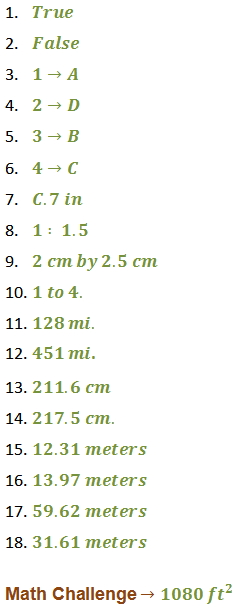Scale Drawing

Scale Drawing

Scale Drawing: the diagram of an object in which the dimensions are proportional to the original object.

Why are scale drawings important?

Careers that involve construction, architecture, city planning, design, and map-making all require knowledge of scale drawings. You also need to be able to use scale drawings when you are traveling (maps).

Using the Scale of a Map

Example

Use the map of Maine to estimate the distance between the towns of China and New Sweden.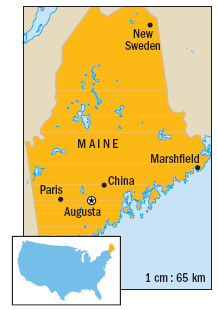Solution

Since there are 4.5 centimeters between China and New Sweden, we can use a proportion to find the distance between the towns.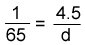Write the proportion to find the distance (d) between towns.d = 654.5 Cross multiply to solve.

d = 292.5

The actual distance between China and New Sweden is about 293 kilometers.

A scale model is very similar to a scale drawing. The scale model is a model of an object with  dimensions that are in proportion to the actual object.

Finding a Dimension on a Scale Model

Example

A scale model of the White House appears in Tobu World Square in Japan. The scale used is 1:25. The height of the main building of the White House is 85 feet. Find this height on the model.

Solution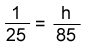Write the proportion to find the height (h) of the model.85 = 25h Cross multiply to solve.

85 = 25h           Divide both sides by 25.
25    25

3.4 = h              Solve.

Answer: The height of the main building of the model is 3.4 feet.

Finding the Scale

Example

A museum is creating a full-size Tyrannosaurus Rex from a model. The model is 40 inches in length from nose to tail. The resulting dinosaur will be 40 feet in length. What is the model's scale?

Solution

Write a ratio in which both measures are in inches, then simplify the fraction.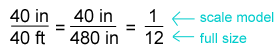Answer: The model's scale is 1:12.

Directions: Are the following statements true or false? Make sure to explain each response.

1. True or False?

The scale on a scale drawing shows how the drawing's dimensions and the actual dimensions of a space are related.

2. True or False?

An object and a scale model of the object are the same size, but they have different shapes.

The scale on a floor plan is 1 in: 23 ft. Match the distance on each floor plan (left column) to each space's actual distance (right column).

 2 in A. 607.2 inches 3.5 in B. 46 feet 2.2 in C. 966 inches 2.6 in D. 59.8 feet

7. You decide to use a scale of 1 in: 7 ft to make a scale drawing of your classroom. If the actual length of your classroom is 49 feet, what should the length of the classroom in the drawing be?

A. 1 in.

B. 42 in.

C. 7 in.

D. 343 in.

8. A child's picnic table is a scale model of an adult picnic table. The child's picnic table is 22 inches tall, and the adult picnic table is 33 inches tall. What is the model's scale?

F.  1 : 22

G. 1 : 15

H. 1 : 1.5

I.  1 : 1

9. An engineer made an 8-inch by 10-inch scale drawing of a computer chip. If the scale on the drawing was 4 inches to 1 centimeter, find the dimensions of the chip.

10. An original 5- by 8-inch photo must be reduced to 1 1/4 by 2 inches to fit in the yearbook. What is the scale of the reduced photo to the original in simplest form?

11. The scale on a map is 1 cm : 32 mi. Find the actual distance in miles for the given length on the map.

4 cm

12. The map scale is 1 cm : 41 mi. Find the actual distance in miles for the given length on the map.

11 cm

13. The map scale is 1 cm : 40 mi. Find the actual distance in miles for the given length on the map.

4.6 cm

14. The map scale is 1 cm : 25 mi. Find the actual distance in miles for the given length on the map.

8.7 cm

15. The scale used to build the models of four airplanes is 1 : 72. Estimate the actual wingspan of the airplane given the wingspan of the model.

Kitty Hawk: 17.1 cm

16. The scale model is 1 : 72. What is the actual wingspan of the real airplane?

Spirit of St. Louis: 19.4 cm

17. The scale model is 1 : 72. What is the actual wingspan of the real airplane?

Boeing 747: 82.8 cm

18. The scale model is 1 : 72. What is the actual wingspan of the real airplane?

Boeing B-17: 43.9 cm